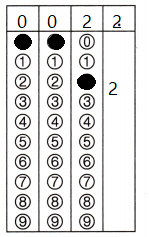Refer to our Texas Go Math Grade 3 Answer Key Pdf to score good marks in the exams. Test yourself by practicing the problems from Texas Go Math Grade 3 Module 12 Assessment Answer Key.

Concepts and Skills

Question 1.
Explain how to find 20 ÷ 4 by making an array. TEKS 3.4.K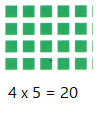Explanation:
20 ÷ 4 = 5
Explained with help of an array

Question 2.
Explain how to find 45 ÷ 5 by using a number line. Show your work on the number line and then explain how you solved the problem. TEKS 3.4.K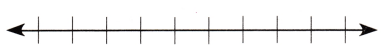Explanation:
The difference between each is 5
45 divide by 5 is 9

Find the unknown factor and quotient. TEKS 3.4.F, 3.4.H, 3.4J, 3.4,K

Question 3.
10 × __ = 50 __ = 50 ÷ 10
10 × 5 = 50
5 = 50 ÷ 10
Explanation:
Quotient = 5

Question 4.
2 × ___ = 16 ___ = 16 ÷ 2
2 × 8 = 16
8 = 16 ÷ 2
Explanation:
Quotient = 8

Question 5.
2 × __ = 20 __ = 20 ÷ 2
2 × 5 = 10
10 = 20 ÷ 2
Explanation:
Quotient = 10

Question 6.
5 × __ = 20 __ = 20 + 5
5 × 4  = 20
4 = 20 ÷ 5
Explanation:
Quotient =  4

Find the quotient. TEKS 3.4F, 3.4.H, 3.4J. 3.4.K

Question 7.
__ = 2 ÷ 2
Explanation:
2 ÷ 2 = 1
Quotient = 1

Question 8.
21 ÷ 3 = ___
Explanation:
21 ÷ 3 = 7
Quotient = 7

Question 9.
__ = 0 ÷ 3
Explanation:
When a number is divided by 0 it is undefined

Question 10.
36 ÷ 4 = __
Explanation:
36 ÷ 4 = 8
Quotient = 8

Question 11.Explanation: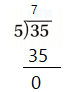Quotient = 7

Question 12.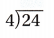Explanation: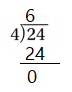Quotient = 6

Question 13.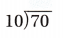Explanation: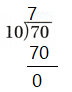Quotient = 7

Question 14.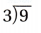Explanation:Quotient = 3

Fill in the bubble for the correct answer choice.
Use division strategies to solve.

Question 15.
Carter has 18 new books. He plans to read 3 of them each week. How many weeks will it take Carter to read
all Of his new books? TEKS3.4.H, 3.4.J,3.4.K
(A) 15 weeks
(B) 6 weeks
(C) 5 weeks
(D) 21 weeks
Explanation:
3 x 6 = 18
6 weeks will it take Carter to read all Of his new book

Question 16.
Gabriella made 5 waffles for breakfast. She has 30 strawberries and 40 blueberries to put on top of the waffles. She will put an equal number of berries on each waffle. How many berries will Gabriella put on each waffle? TEKS 3.4.H, 3.4.K, 3.5.B
(A) 12
(B) 8
(C) 14
(D) 6
Explanation:
Gabriella made 5 waffles for breakfast.
She has 30 strawberries and 40 blueberries to put on top of the waffles.
14 berries will Gabriella put on each waffle

Question 17.
There are 40 people at the fair waiting in line for a ride. Each car in the ride can hold 10 people. Which equation could be used to find the number of cars needed to hold all 40 people? TEKS 3.4.H, 3.4.J, 3.4.K, 3.5.B
(A) 40 – 10 = ___
(B) 40 ÷ 10 = ___
(C) __ + 10 = 40
(D) 40 × 10 = __
Explanation:
There are 40 people at the fair waiting in line for a ride.
Each car in the ride can hold 10 people.
40 ÷ 10 = 4  equation could be used to find the number of cars needed to hold all 40 people

Question 18.
Alyssa has 4 cupcakes. She gives 2 cupcakes to each of her cousins. How many cousins does Alyssa have? TEKS 3.4.H, 3.4.K, 3.5.B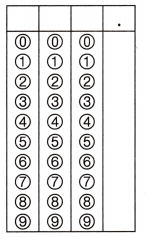Record your answer and fill in the bubbles on the grid. Be sure to use the correct place value.